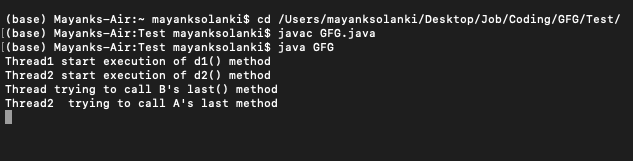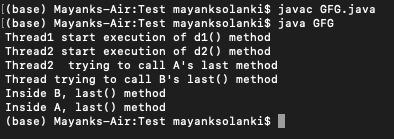• Last Updated : 15 Jun, 2022

If two threads are waiting for each other forever such type of infinite waiting is called deadlock in java. Synchronized keyword is the only reason for deadlock situation hence while using synchronized keyword we have to take special care. There is no resolution technique for deadlock, but several prevention techniques are available.

Example 1:

## Java

 `// Java program to illustrate Deadlock``// where deadlock occurs` `// Importing required packages``import` `java.io.*;``import` `java.util.*;` `// Class 1``// Helper class``class` `A {` `    ``// Method 1 of this class``    ``// Synchronized method``    ``public` `synchronized` `void` `last()``    ``{` `        ``// Print and display statement``        ``System.out.println(``"Inside A, last() method"``);``    ``}` `    ``// Method 2 of this class``    ``// Synchronized method``    ``public` `synchronized` `void` `d1(B b)``    ``{``        ``System.out.println(``            ``"Thread1 start execution of d1() method"``);` `        ``// Try block to check for exceptions``        ``try` `{` `            ``// Putting the current thread to sleep for``            ``// specific time using sleep() method``            ``Thread.sleep(``2000``);``        ``}` `        ``// Catch block to handle the exceptions``        ``catch` `(InterruptedException e) {``            ``// Display the exception on the console``            ``System.out.println(e);``        ``}` `        ``// Display statement``        ``System.out.println(``            ``"Thread trying to call B's last() method"``);` `        ``// Calling the method 1 of this class as created``        ``// above``        ``b.last();``    ``}``}` `// Class 2``// Helper class B``class` `B {` `    ``// Method 1 of this class``    ``public` `synchronized` `void` `last()``    ``{` `        ``// Display statement only``        ``System.out.println(``"Inside B, last() method"``);``    ``}` `    ``// Method 2 of this class``    ``// Synchronized the method d2``    ``public` `synchronized` `void` `d2(A a)``    ``{` `        ``// Display message only``        ``System.out.println(``            ``"Thread2 start execution of d2() method"``);` `        ``// Try block to check for exceptions``        ``try` `{` `            ``// Putting the current thread to sleep for``            ``// certain time using sleep() method``            ``Thread.sleep(``2000``);` `            ``// Catch block to handle the exceptions``        ``}``        ``catch` `(InterruptedException e) {` `            ``// Display the exception on the console``            ``System.out.println(e);``        ``}` `        ``// Display message only``        ``System.out.println(``            ``"Thread2  trying to call A's last method"``);` `        ``// Again calling the last() method inside this class``        ``a.last();``    ``}``}` `// Class 3``// Main class``// Deadlock class which is extending Thread class``class` `GFG ``extends` `Thread {` `    ``// Creating object of type class A``    ``A a = ``new` `A();` `    ``// Creating object of type class B``    ``B b = ``new` `B();` `    ``// Method 1``    ``public` `void` `m1()``    ``{` `        ``// Starting the thread``        ``this``.start();` `        ``// Calling d1 method of class A``        ``a.d1(b);``    ``}` `    ``// Method 2``    ``// run() method for the thread``    ``public` `void` `run()``    ``{` `        ``// Calling d2 method of class B``        ``b.d2(a);``    ``}` `    ``// Method 3``    ``// Main driver method``    ``public` `static` `void` `main(String[] args)``    ``{` `        ``// Creating object of this class``        ``GFG deadlock = ``new` `GFG();` `        ``// Calling m1 method``        ``deadlock.m1();``    ``}``}`

Output:Output explanation:

Here the cursor is showing forever because the threads enter into the deadlock situation. In the above program if we removed at least one synchronized keyword then the program won’t enter into the deadlock situation. Hence, synchronized keyword is one of the major reason for deadlock situation. Due to this while using synchronized keyword we have to take special care.

We can avoid Deadlock situation in the following ways:

• Using Thread.join() Method: We can get a deadlock if two threads are waiting for each other to finish indefinitely using thread join. Then our thread has to wait for another thread to finish, it is always best to use Thread.join() method with the maximum time you want to wait for the thread to finish.
• Use Lock Ordering: We have to always assign a numeric value to each lock and before acquiring the lock with a higher numeric value we have to acquire the locks with a lower numeric value.
• Avoiding unnecessary Locks: We should use locks only for those members on which it is required, unnecessary use of locks leads to a deadlock situation. And it is recommended to use a lock-free data structure and If it is possible to keep your code free from locks. For example, instead of using synchronized ArrayList use the ConcurrentLinkedQueue.

## Java

 `// Java program to illustrate Deadlock``// where deadlock is prevented from occurring` `// Importing required packages``import` `java.io.*;``import` `java.util.*;` `// Class 1``// Helper class``class` `A {` `    ``// Method 1 of this class``    ``// Synchronized method``    ``public` `synchronized` `void` `last()``    ``{` `        ``// Print and display statement``        ``System.out.println(``"Inside A, last() method"``);``    ``}` `    ``// Method 2 of this class``    ``// Synchronized method``    ``public` `synchronized` `void` `d1(B b)``    ``{``        ``System.out.println(``            ``"Thread1 start execution of d1() method"``);` `        ``// Try block to check for exceptions``        ``try` `{` `            ``// Putting the current thread to sleep for``            ``// specific time using sleep() method``            ``Thread.sleep(``2000``);``        ``}` `        ``// Catch block to handle the exceptions``        ``catch` `(InterruptedException e) {``            ``// Display the exception on the console``            ``System.out.println(e);``        ``}` `        ``// Display statement``        ``System.out.println(``            ``"Thread trying to call B's last() method"``);` `        ``// Calling the method 1 of this class as created``        ``// above``        ``b.last();``    ``}``}` `// Class 2``// Helper class B``class` `B {` `    ``// Method 1 of this class``    ``public` `void` `last()``    ``{` `        ``// Display statement only``        ``System.out.println(``"Inside B, last() method"``);``    ``}` `    ``// Method 2 of this class``    ``// Non-synchronized the method d2``    ``public` `void` `d2(A a)``    ``{` `        ``// Display message only``        ``System.out.println(``            ``"Thread2 start execution of d2() method"``);` `        ``// Try block to check for exceptions``        ``try` `{` `            ``// Putting the current thread to sleep for``            ``// certain time using sleep() method``            ``Thread.sleep(``2000``);` `            ``// Catch block to handle the exceptions``        ``}``        ``catch` `(InterruptedException e) {` `            ``// Display the exception on the console``            ``System.out.println(e);``        ``}` `        ``// Display message only``        ``System.out.println(``            ``"Thread2  trying to call A's last method"``);` `        ``// Again calling the last() method inside this class``        ``a.last();``    ``}``}` `// Class 3``// Main class``// Deadlock class which is extending Thread class``class` `GFG ``extends` `Thread {` `    ``// Creating object of type class A``    ``A a = ``new` `A();` `    ``// Creating object of type class B``    ``B b = ``new` `B();` `    ``// Method 1``    ``public` `void` `m1()``    ``{` `        ``// Starting the thread``        ``this``.start();` `        ``// Calling d1 method of class A``        ``a.d1(b);``    ``}` `    ``// Method 2``    ``// run() method for the thread``    ``public` `void` `run()``    ``{` `        ``// Calling d2 method of class B``        ``b.d2(a);``    ``}` `    ``// Method 3``    ``// Main driver method``    ``public` `static` `void` `main(String[] args)``    ``{` `        ``// Creating object of this class``        ``GFG deadlock = ``new` `GFG();` `        ``// Calling m1 method``        ``deadlock.m1();``    ``}``}`

Output:My Personal Notes arrow_drop_up